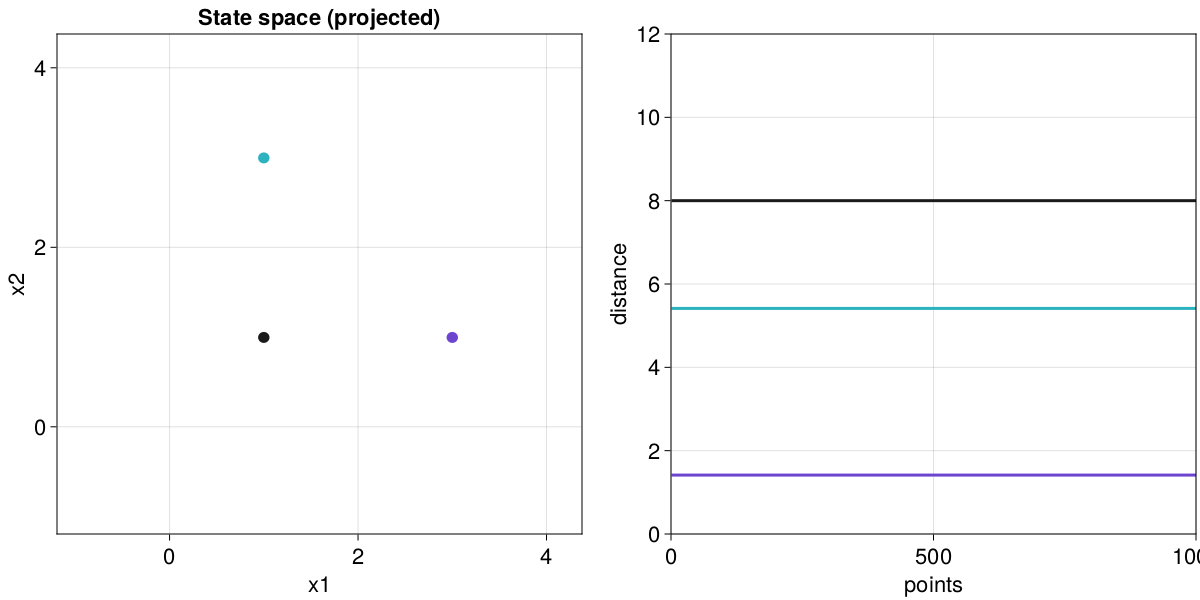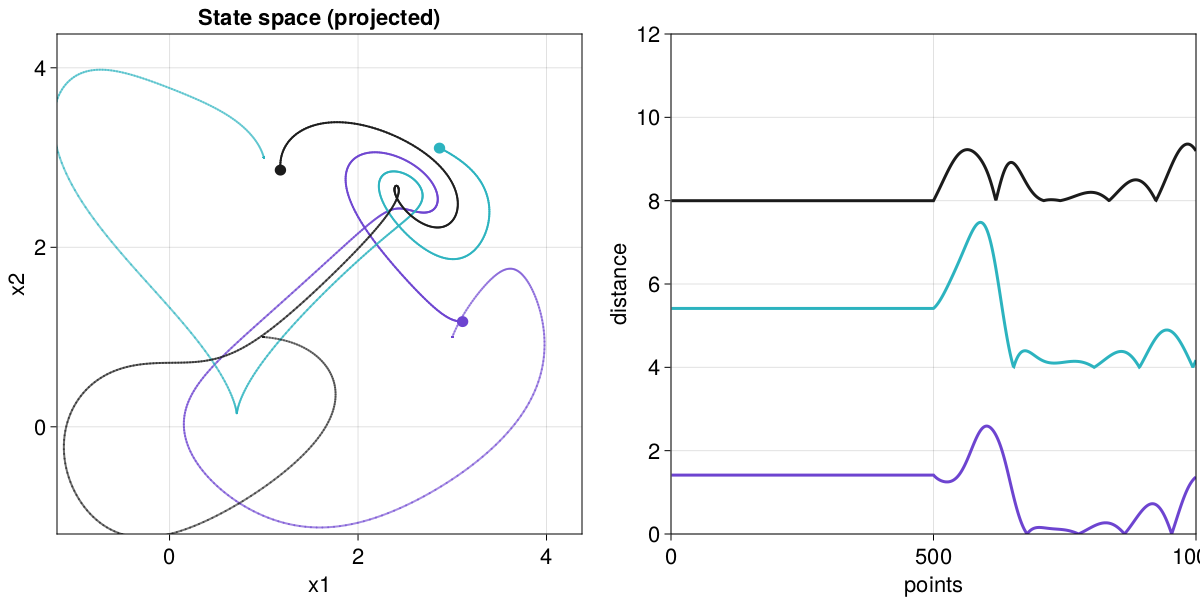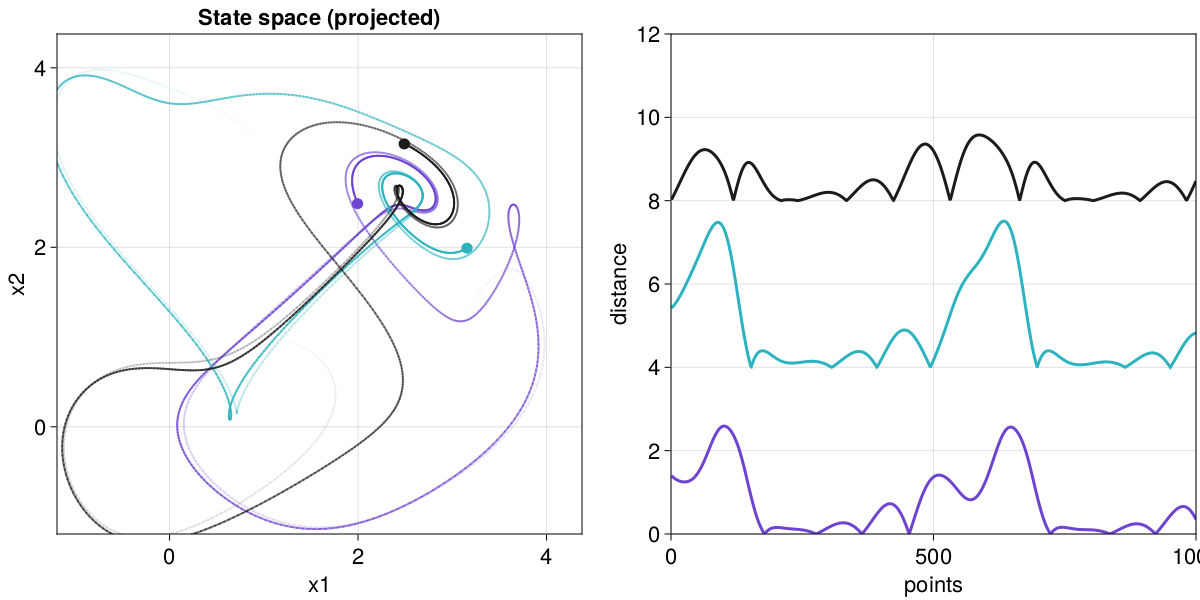# Interactive GUIs for Dynamical Systems

Via the package InteractiveDynamics.jl we have created several GUI applications for exploring dynamical systems which are integrated with DynamicalSystems.jl. The GUI apps use the Makie ecosystem, and have been designed to favor generality and simple source code. This means that even if one of the available GUI apps does not do what you'd like to do, it should be easy to copy its source code and adjust accordingly!

This documentation page is built using versions:

using Pkg
Pkg.status(["DynamicalSystems", "InteractiveDynamics"];
mode = PKGMODE_MANIFEST, io=stdout
)
Status ~/work/DynamicalSystems.jl/DynamicalSystems.jl/docs/Manifest.toml
 DynamicalSystems v2.3.2 ~/work/DynamicalSystems.jl/DynamicalSystems.jl
[ec714cd0] InteractiveDynamics v0.21.11

## Interactive Trajectory Evolution

InteractiveDynamics.interactive_evolutionFunction
interactive_evolution(ds::DynamicalSystem [, u0s]; kwargs...) → fig, obs, step

Launch an interactive GUI application that can evolve the initial conditions u0s (vector of vectors) of the given dynamical system. All initial conditions are evolved in parallel and at exactly the same time.

Added controls allow you to step/run/pause the evolution and to control after how many integrator steps the plots are updated. The application can run forever (trajectories are computed on demand).

By default the GUI window displays statespace and timeseries plots. It also allows changing the parameters of ds live during the system evolution, see keyword ps below in "Parameter Keywords".

The function returns fig, obs, step, paramvals. fig is the overarching figure (the entire GUI) and can be recorded with Makie.record. obs is a vector of observables, each containing the current state of each trajectory. step is an observable that triggers the stepping of each trajectory and the update of the plots. Do step[] = 0 (or any other integer), to trigger an update. paramvals is an observable containing current parameter values, and is only valid if ps is not nothing, see keyword ps below in "Parameter Keywords".

The figure layout is as follows:

1. fig[1,1] = state space plot (fig[1,1][1,1]) and time evolution controls
2. fig[1,2] = timeseries plots
3. fig[2,:] = parameter controls (if ps is given)

This means that you can make any kind of composite plots and videos starting from the figure returned from interactive_evolution. See the documentation online for more such examples.

State Space Keywords

• transform = identity: Transformation applied to the state of the dynamical system before plotting. Can even return a vector that is of higher dimension than ds.
• idxs = 1:min(length(transform(u0s)), 3): Which variables to plot (up to three can be chosen). Variables are selected after transform has been applied.
• colors: The color for each initial condition (and resulting trajectory).
• lims: A tuple of tuples (min, max) for the axis limits. If not given, they are automatically deduced by evolving each of u0s 1000 units and picking most extreme values (limits are very hard to adjust after application is launched).
• m = 1.0: The trajectory endpoints have a marker. A heuristic is done to choose appropriate marker size given the trajectory size. m is a multiplier that scales the marker size.
• tail = 1000: Length of plotted trajectory (in step units of the integrator).
• fade = true: For continuous time system, the trajectories in state space are faded to full transparency if true.
• plotkwargs = NamedTuple() : A named tuple of keyword arguments propagated to the state space plot (lines for continuous, scatter for discrete systems). plotkwargs can also be a vector of named tuples, in which case each initial condition gets different arguments.
• diffeq = NamedTuple(): Named tuple of keyword arguments propagated to the solvers of DifferentialEquations.jl (for continuous systems). Because trajectories are not pre-computed and interpolated, but rather calculated on the fly step by step, it is strongly recommended to use an ODE solver thas has a constant step size instead of being adaptive. For example diffeq = (alg=Tsit5(), adaptive=false, dt=0.01).
• add_controls = true: Whether to add buttons and sliders for interactively controlling the trajectory evolution. Should be false only if composite videos are intended to be produced using the returned step. If false, the keyword steps_per_update = 1 decides how many steps to take before updating plots.

Timeseries Keywords

• tsidxs = idxs: Indices selecting variables to be plotted as timeseries. You can pass nothing instead and no timeseries will be plotted.
• total_span: How much the x-axis of the timeseries plots should span (in real time units)
• linekwargs = NamedTuple(): Extra keywords propagated to the timeseries plots.

Parameter Keywords

• ps = nothing: If ps is not nothing, then it must be a dictionary, mapping keys of the system parameter container (ds.p) to possible ranges of values. The app then will add some additional controls on the bottom of the GUI which allow one to interactively change system parameters and then click the "update" button to translate the new parameters to system evolution. This can be done without stopping the live system evolution. Notice that in this scenario it is recommended to provide the lims keyword manually. An extra argument is returned in this case: a dictionary mapping parameter keys to observables containing their current values. You can use this to generate additional plot elements that may depend on system parameters and thus need to be changed if the sliders are changed.
• pnames = Dict(keys(ps) .=> keys(ps)) : Dictionary mapping parameter keys to labels. Only valid if params is a dictionary and not nothing.

In addition the keywords figure, axis can be named tuples with arbitrary keywords propagated to the generation of the Figure and state space Axis instances.

For example, the animation on the top of this section was done with:

using InteractiveDynamics
using DynamicalSystems, GLMakie
using OrdinaryDiffEq

diffeq = (alg = Tsit5(), adaptive = false, dt = 0.01)
ps = Dict(
1 => 1:0.1:30,
2 => 10:0.1:50,
3 => 1:0.01:10.0,
)
pnames = Dict(1 => "σ", 2 => "ρ", 3 => "β")

lims = (
(-30, 30),
(-30, 30),
(0, 100),
)

ds = Systems.lorenz()

u1 = [10,20,40.0]
u3 = [20,10,40.0]
u0s = [u1, u3]

idxs = (1, 2, 3)
diffeq = (alg = Tsit5(), dt = 0.01, adaptive = false)

figure, obs, step, slidervals = interactive_evolution(
ds, u0s; ps, idxs, tail = 1000, diffeq, pnames, lims
)

# Use the slidervals observable to plot fixed points
lorenzfp(ρ,β) = [
Point3f(sqrt(β*(ρ-1)), sqrt(β*(ρ-1)), ρ-1),
Point3f(-sqrt(β*(ρ-1)), -sqrt(β*(ρ-1)), ρ-1),
]

fpobs = lift(lorenzfp, slidervals, slidervals)
ax = content(figure[1,1][1,1])
scatter!(ax, fpobs; markersize = 20, marker = :diamond, color = :black)

Notice that the last part of the code plots the fixed points of the system (something interactive_evolution does not do by itself), and the fixed points plots are automatically updated when a parameter is changed in the GUI, because it uses the observable paramvals.

### Customized animations

It is straightforward to add custom plots and generate extra animations from the interface of the step observable returned by interactive_evolution. In the following example we'll make a video that rotates around some interlaced periodic trajectories, and plot some stuff from them on an extra panel to the right.

using DynamicalSystems, InteractiveDynamics, CairoMakie
using OrdinaryDiffEq: Tsit5
using LinearAlgebra: dot, norm

ds = Systems.thomas_cyclical(b = 0.2)
u0s = ([3, 1, 1.], [1, 3, 1.], [1, 1, 3.])
diffeq = (alg = Tsit5(), adaptive = false, dt = 0.05)

fig, obs, step, = interactive_evolution(
ds, u0s; tail = 1000, diffeq, add_controls = false, tsidxs = nothing,
# Replace this with [1, 2, 3] if using GLMakie (looks much cooler ;))
idxs = [1, 2],
figure = (resolution = (1200, 600),),
)
axss = content(fig[1,1][1,1])
axss.title = "State space (projected)"

# Plot some stuff on a second axis that use obs
# Plot distance of trajectory from symmetry line
ax = Axis(fig[1,1][1,2]; xlabel = "points", ylabel = "distance")
function distance_from_symmetry(u)
v = 0*SVector(u...) .+ 1/√(length(u))
t = dot(v, u)
return norm(u - t*v)
end
for (i, ob) in enumerate(obs)
y = lift(x -> distance_from_symmetry.(x) .+ 4(i-1), ob)
x = 1:length(y[])
lines!(ax, x, y; color = JULIADYNAMICS_COLORS[i])
end
ax.limits = ((0, 1000), (0, 12))
figNow we can step this animation arbitrarily many times

for j in 1:500; step[] = 0; end
figfor j in 1:500; step[] = 0; end
figOr we could produce a video with:

record(fig, "thomas_cyclical.mp4"; framerate = 60) do io
for i in 1:720
recordframe!(io)
# Step multiple times per frame for "faster" animation
for j in 1:5; step[] = 0; end
end
end

## Cobweb Diagrams

InteractiveDynamics.interactive_cobwebFunction
interactive_cobweb(ds::DiscreteDynamicalSystem, prange, O::Int = 3; kwargs...)

Launch an interactive application for exploring cobweb diagrams of 1D discrete dynamical systems. Two slides control the length of the plotted trajectory and the current parameter value. The parameter values are obtained from the given prange.

In the cobweb plot, higher order iterates of the dynamic rule f are plotted as well, starting from order 1 all the way to the given order O. Both the trajectory in the cobweb, as well as any iterate f can be turned off by using some of the buttons.

Keywords

• fkwargs = [(linewidth = 4.0, color = randomcolor()) for i in 1:O]: plotting keywords for each of the plotted iterates of f
• trajcolor = :black: color of the trajectory
• pname = "p": name of the parameter slider
• pindex = 1: parameter index
• xmin = 0, xmax = 1: limits the state of the dynamical system can take
• Tmax = 1000: maximum trajectory length
• x0s = range(xmin, xmax; length = 101): Possible values for the x0 slider.

The animation at the top of this section was done with

using InteractiveDynamics, GLMakie, DynamicalSystems

# the second range is a convenience for intermittency example of logistic
rrange = 1:0.001:4.0
# rrange = (rc = 1 + sqrt(8); [rc, rc - 1e-5, rc - 1e-3])

lo = Systems.logistic(0.4; r = rrange)
interactive_cobweb(lo, rrange, 5)

## Orbit Diagrams

Notice that orbit diagrams and bifurcation diagrams are different things in DynamicalSystems.jl

InteractiveDynamics.interactive_orbitdiagramFunction
interactive_orbitdiagram(
ds::DiscreteDynamicalSystem, p_index, pmin, pmax, i0 = 1;
u0 = get_state(ds), parname = "p", title = ""
)

Open an interactive application for exploring orbit diagrams (ODs) of discrete dynamical systems. Requires DynamicalSystems.

Keywords control the name of the parameter, the initial state (used for any parameter) or whether to add a title above the orbit diagram.

Interaction

The application is separated in the "OD plot" (left) and the "control panel" (right). On the OD plot you can interactively click and drag with the left mouse button to select a region in the OD. This region is then re-computed at a higher resolution.

The options at the control panel are straight-forward, with

• n amount of steps recorded for the orbit diagram (not all are in the zoomed region!)
• t transient steps before starting to record steps
• d density of x-axis (the parameter axis)
• α alpha value for the plotted points.

Notice that at each update n*t*d steps are taken. You have to press update after changing these parameters. Press reset to bring the OD in the original state (and variable). Pressing back will go back through the history of your exploration History is stored when the "update" button is pressed or a region is zoomed in.

You can even decide which variable to get the OD for by choosing one of the variables from the wheel! Because the y-axis limits can't be known when changing variable, they reset to the size of the selected variable.

Accessing the data

What is plotted on the application window is a true orbit diagram, not a plotting shorthand. This means that all data are obtainable and usable directly. Internally we always scale the orbit diagram to [0,1]² (to allow Float64 precision even though plotting is Float32-based). This however means that it is necessary to transform the data in real scale. This is done through the function scaleod which accepts the 5 arguments returned from the current function:

figure, oddata = interactive_orbitdiagram(...)
ps, us = scaleod(oddata)

The animation at the top of this section was done with

i = p_index = 1
ds, p_min, p_max, parname = Systems.henon(), 0.8, 1.4, "a"
t = "orbit diagram for the Hénon map"

oddata = interactive_orbitdiagram(ds, p_index, p_min, p_max, i;
parname = parname, title = t)

ps, us = scaleod(oddata)

## Interactive Poincaré Surface of Section

InteractiveDynamics.interactive_poincaresosFunction
interactive_poincaresos(cds, plane, idxs, complete; kwargs...)

Launch an interactive application for exploring a Poincaré surface of section (PSOS) of the continuous dynamical system cds. Requires DynamicalSystems.

The plane can only be the Tuple type accepted by DynamicalSystems.poincaresos, i.e. (i, r) for the ith variable crossing the value r. idxs gives the two indices of the variables to be displayed, since the PSOS plot is always a 2D scatterplot. I.e. idxs = (1, 2) will plot the 1st versus 2nd variable of the PSOS. It follows that plane ∉ idxs must be true.

complete is a three-argument function that completes the new initial state during interactive use, see below.

The function returns: figure, laststate with the latter being an observable containing the latest initial state.

Keyword Arguments

• direction, rootkw : Same use as in DynamicalSystems.poincaresos.
• tfinal = (1000.0, 10.0^4) : A 2-element tuple for the range of values for the total integration time (chosen interactively).
• color : A function of the system's initial condition, that returns a color to plot the new points with. The color must be RGBf/RGBAf. A random color is chosen by default.
• labels = ("u₁" , "u₂") : Scatter plot labels.
• scatterkwargs = (): Named tuple of keywords passed to scatter.
• diffeq = NamedTuple() : Any extra keyword arguments are passed into init of DiffEq.

Interaction

The application is a standard scatterplot, which shows the PSOS of the system, initially using the system's u0. Two sliders control the total evolution time and the size of the marker points (which is always in pixels).

Upon clicking within the bounds of the scatter plot your click is transformed into a new initial condition, which is further evolved and its PSOS is computed and then plotted into the scatter plot.

Your click is transformed into a full D-dimensional initial condition through the function complete. The first two arguments of the function are the positions of the click on the PSOS. The third argument is the value of the variable the PSOS is defined on. To be more exact, this is how the function is called:

x, y = mouseclick; z = plane
newstate = complete(x, y, z)

The complete function can throw an error for ill-conditioned x, y, z. This will be properly handled instead of breaking the application. This newstate is also given to the function color that gets a new color for the new points.

To generate the animation at the start of this section you can run

using InteractiveDynamics, GLMakie, OrdinaryDiffEq, DynamicalSystems
diffeq = (alg = Vern9(), abstol = 1e-9, reltol = 1e-9)

hh = Systems.henonheiles()

potential(x, y) = 0.5(x^2 + y^2) + (x^2*y - (y^3)/3)
energy(x,y,px,py) = 0.5(px^2 + py^2) + potential(x,y)
const E = energy(get_state(hh)...)

function complete(y, py, x)
V = potential(x, y)
Ky = 0.5*(py^2)
Ky + V ≥ E && error("Point has more energy!")
px = sqrt(2(E - V - Ky))
ic = [x, y, px, py]
return ic
end

plane = (1, 0.0) # first variable crossing 0

# Coloring points using the Lyapunov exponent
function λcolor(u)
λ = lyapunovs(hh, 4000; u0 = u)
λmax = 0.1
return RGBf(0, 0, clamp(λ/λmax, 0, 1))
end

state, scene = interactive_poincaresos(hh, plane, (2, 4), complete;
labels = ("q₂" , "p₂"),  color = λcolor, diffeq...)

## Scanning a Poincaré Surface of Section

InteractiveDynamics.brainscan_poincaresosFunction
brainscan_poincaresos(A::Dataset, j::Int; kwargs...)
brainscan_poincaresos(As::Vector{Dataset}, j::Int; kwargs...)

Launch an interactive application for scanning a Poincare surface of section of A like a "brain scan", where the plane that defines the section can be arbitrarily moved around via a slider. Return figure, ax3D, ax2D.

The input dataset must be 3 dimensional, and here the crossing plane is always chosen to be when the j-th variable of the dataset crosses a predefined value. The slider automatically gets all possible values the j-th variable can obtain.

If given multiple datasets, the keyword colors attributes a color to each one, e.g. colors = [JULIADYNAMICS_COLORS[mod1(i, 6)] for i in 1:length(As)].

The keywords linekw, scatterkw are named tuples that are propagated as keyword arguments to the line and scatter plot respectively, while the keyword direction = -1 is propagated to the function DyamicalSystems.poincaresos.

using GLMakie, DynamicalSystems, InteractiveDynamics
using OrdinaryDiffEq

ds = Systems.henonheiles()
diffeq = (alg = Vern9(),)
u0s = [
[0.0, -0.25, 0.42081, 0.0],
[0.0, 0.1, 0.5, 0.0],
[0.0, -0.31596, 0.354461, 0.0591255]
]
trs = [trajectory(ds, 10000, u0; diffeq)[:, SVector(1,2,3)] for u0 ∈ u0s]
for i in 2:length(u0s)
append!(trs, trs[i])
end

# Inputs:
j = 2 # the dimension of the plane
tr = trs

brainscan_poincaresos(tr, j; linekw = (transparency = true,))